# ski-in / ski-out - Page 17

Viewing mode
656 results
•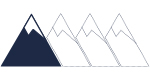• Slopes access :
• ski-in (-50 m)
• Rooms :
• Studio
• Studio + cabin(s)
• Total surface (sq.m) :
• 33 sq.m
• Garage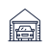•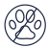• Slopes access :
• 50 -300m to the slopes
• Rooms :
• 3 rooms
• Total surface (sq.m) :
• 40 sq.m
•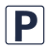•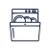•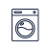•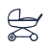•• Slopes access :
• ski-in (-50 m)
• Rooms :
• 4 rooms
•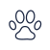•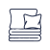•••• Slopes access :
• 50 -300m to the slopes
• Rooms :
• 4 rooms
• 4 rooms + cabin(s)
• Total surface (sq.m) :
• 88 sq.m
••••• Slopes access :
• 50 -300m to the slopes
• Rooms :
• Studio
• Studio + cabin(s)
• Total surface (sq.m) :
• 32 sq.m
•••• Slopes access :
• ski-in (-50 m)
• Rooms :
• Studio
• Studio + cabin(s)
• Total surface (sq.m) :
• 31 sq.m
••••• Slopes access :
• 50 -300m to the slopes
• Rooms :
• 6 rooms
• Total surface (sq.m) :
• 250 sq.m
•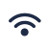••• Garage••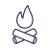•••• Slopes access :
• 50 -300m to the slopes
• Rooms :
• 2 rooms
• 2 rooms + cabin(s)
• Total surface (sq.m) :
• 38 sq.m
••••• Slopes access :
• ski-in (-50 m)
• Rooms :
• 2 rooms
• 2 rooms + cabin(s)
• Total surface (sq.m) :
• 45 sq.m
•••••• Slopes access :
• 50 -300m to the slopes
• Total surface (sq.m) :
• 58 à 75 sq.m
•••••• Slopes access :
• ski-in (-50 m)
• Rooms :
• Studio
• Studio + cabin(s)
• Total surface (sq.m) :
• 31 sq.m
••• Slopes access :
• 50 -300m to the slopes
• Rooms :
• Duplex
• Total surface (sq.m) :
• 110 sq.m
•••••• Slopes access :
• ski-in (-50 m)
• Rooms :
• Studio
• Studio + cabin(s)
• Total surface (sq.m) :
• 21 sq.m
••• Slopes access :
• ski-in (-50 m)
• Rooms :
• Studio
• Studio + cabin(s)
• Total surface (sq.m) :
• 27 sq.m
•••• Slopes access :
• 50 -300m to the slopes
• Rooms :
• 3 rooms
• 3 rooms + cabin(s)
• Total surface (sq.m) :
• 62 sq.m
•••••• Slopes access :
• ski-in (-50 m)
• Rooms :
• Studio
• Studio + cabin(s)
• Total surface (sq.m) :
• 30 sq.m
•••••• Slopes access :
• ski-in (-50 m)
• Rooms :
• Studio
• Studio + cabin(s)
• Total surface (sq.m) :
• 34 sq.m
••• Slopes access :
• ski-in (-50 m)
• Rooms :
• Duplex
• Total surface (sq.m) :
• 64 sq.m
•••••• Slopes access :
• 50 -300m to the slopes
• Rooms :
• Studio
• Total surface (sq.m) :
• 16 sq.m
•• Slopes access :
• ski-in (-50 m)
• Rooms :
• Studio
• Studio + cabin(s)
• Total surface (sq.m) :
• 29 sq.m
•••